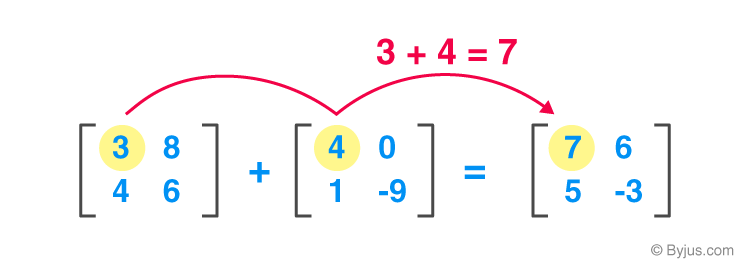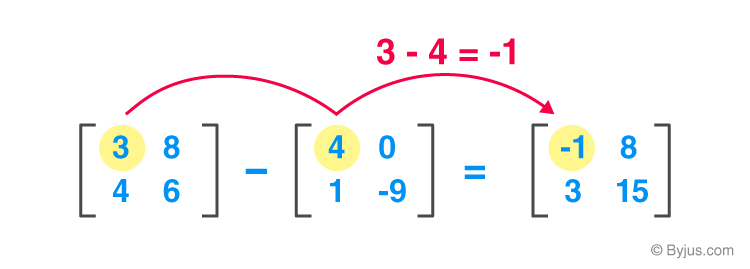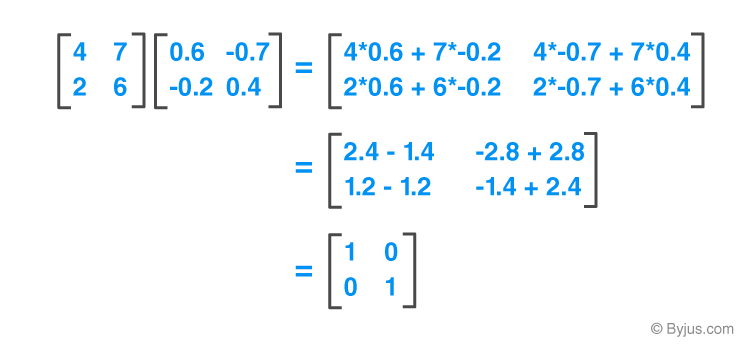Jet Set Go! All about Aeroplanes Jet Set Go! All about Aeroplanes

# Application Of Matrices

The application of matrix plays a major role in Mathematics, as well as in other fields. It helps in solving linear equations. Matrices are extremely valuable objects that can be found in a wide range of applications. The application of matrices in mathematics is used in a wide range of scientific fields as well as mathematical areas. Engineering mathematics is used in almost every aspect of our lives. In this article, we are going to learn what is a matrix, different matrix operations and the application of matrices in detail.

## What is a Matrix?

The matrix in mathematics is a rectangular or square array of numbers or variables, arranged in the form of rows and columns. Individual items in a matrix are known as elements or entries.

The size of the matrix is determined by some its rows and columns. Matrix with ‘m’ rows and ‘n’ columns is read as ‘m*n’ matrix where m and n are its dimensions.

$$\begin{array}{l}A=\begin{bmatrix} 1 & 2 & 3 & 4\\ 5 & 6 & 7 & 8\\ 9 & 10 & 11 & 12 \end{bmatrix}\end{array}$$

For example ,the matrix A mentioned above  is a 3*4 matrix ,where 1,5,9,2,6 etc are its elements.

## Matrix Operations

Two matrices can be added or subtracted element by element, provided both are of the same size.

The below image will help us in understanding the addition and subtraction operation on matrices.### Subtraction of Matrices### Matrix Multiplication

There is a rule for matrix multiplication, the number of columns in the first matrix should be equal to the number of rows in the second.

If A is a matrix of m*n and B is a matrix of n*p then their product matrix C=(A*B) will be m*p, whose elements are produced by the dot product of a corresponding row of A and a corresponding column of B.

The below image gives us a better understanding of the multiplication of matrices.## Applications of Matrices in Various Fields

The application of Matrices in different fields are explained below:

### Application of Matrices in Cryptography

Cryptography is the process of encrypting data so that only the appropriate individual has access to it and can draw conclusions. The process of encryption is carried out with the help of an invertible key. In this method, matrices are used. Digital audio or video signal is first viewed as a series of integers that indicate an acoustic audio signal’s variation in air pressure over time. In this method, filtering techniques are employed, which are based on matrix multiplication.

### Application of Matrices in Wireless Communication

Wireless signals are modelled and optimized using matrices. Matrixes are used to detect, extract, and process the information encoded in signals. The estimation of signals and detecting problems on wireless communication heavily relies on matrices. We all know that a significant part of the telecommunication business is wireless communication. We can find the application of matrices over here, as it is useful for processing and displaying digital images.

### Application of Matrices in Computer Graphics

A square matrix is a simple way to represent linear object transformations. In the realm of graphics, matrices are used to project three-dimensional images into two-dimensional planes. To begin with, a digital image is treated as a matrix in graphics. The matrix’s rows and columns correspond to the rows and columns of pixels, and the numerical entries correspond to the colour values of the pixels. In video game graphics, manipulating a point with matrices is a typical mathematical strategy. Graphs are also expressed using matrices.

Apart from these applications, matrices are used in Fourier analysis, Gauss theorem, Finding electric currents using matrix equations, finding forces in the bridge. This is all about the application of matrices.

Stay tuned with BYJU’S – The Learning App and download the app to learn all Maths-concepts easily by exploring more videos.